# Percentage Concept

## Percentage Concept

### Basic Definition:

• The term percent comes from the Latin phrase ‘per centum’ which means per hundred or for every hundred. It is a fraction whose denominator is 100 and numerator is percent, e.g.• In mathematics, the percentage is denoted by the symbol ‘%’.
• Percentages are fractions with a denominator of 100.
• To convert a percentage to its fraction equivalent, we begin by writing it with a denominator of 100. We can then simplify.
E.g.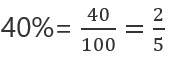• The percentage of a given number ‘n’ is given by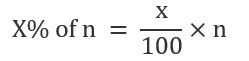Examples: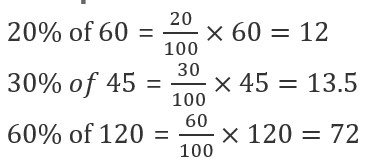### Converting Fractions to Percentage

A fraction can be represented byTo convert a fraction into a percentage, we will simply multiply the fraction with 100%

E.g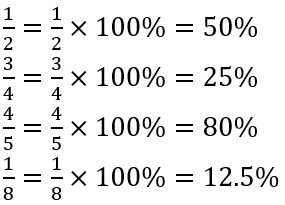### Percentage Increase

When some value increase over time, and we want to calculate the percentage increase, we first find the difference between the original value and the increased value.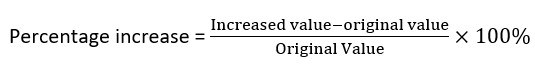Example. The population of a town is 15,000. After 1 year the population of the town becomes 16500. Find the percentage increase in the population?

Solutions. Using formula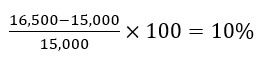So, the population is increased by 10%

If something is increase by a% then it becomes (100+a)% of itself

Example. Amit weight is 50Kg. After 1 year his weight is increased by 20% then find its new weight

Solutions. Amit weight = 50 kg
Increase in weight = 20% of 50 = 10 kg
Final Weight = 50+10 = 60 kg

Or, it becomes ( 100+ 20 ) % of 50 = 120% of 50 = (120/100) x 50 = 60

Example. Population of a town is 12,000. If the population of town is increase by 30%, then find the population after 1 year ?
Solution. The population is increased by 30%, so new populations become 130% of the initial population which is 12,000
Now, New population = 130% of 12,000 = 15,600

Or,  Increase in population = 30% of 12,000 = 3,600
Total pollution = 12,000+3,600 = 15,600

### Percentage Decrease

When some value in decreases over time, and we want to calculate the percentage decreases, we first find the difference between the original value and the decreased value.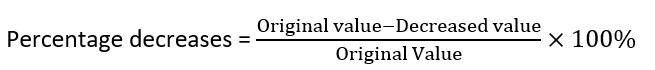Example. The population of a town is 15,000. After 1 year the population of the town becomes 12,000. Find the percentage decreases in the population?

Solutions.Using formulaSo, the population is decreased by 20%

If something is decreased by a% then it becomes (100-a)% of itself

Example Ram weight is 40Kg. After 1 year his weight is Decreased by 20% then find its new weight.
Solutions. Ram weight = 40 Kg
Decrease in weight = 20% of 40 = 8 kg
Final Weight = 40-8 = 32 kg

Or,  it becomes ( 100- 20 ) % of 40 = 80% of 40 = 32 Kg

Example Population of a town is 12,000. If the population of town is decrease by 30%, then find the population after 1 year.
Solutions.Population is decreased by 30%, so new pollutions becomes (100-30)% = 70% of initial population which is 12,000
Now, New population = 70% of 12,000 = 8,400

Or,  Decrease in population = 30% of 12,000 = 3,600
Total pollution = 12,000-3,600 = 8,400

For feedback and Query Mail us at contact@ambitiousbaba.com